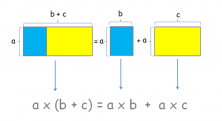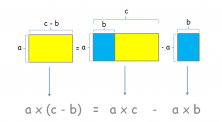Smartick is an advanced online program that teaches kids math and coding in only 15 min. a day

Sep27

# Learn about the Distributive Property of Multiplication

In today’s entry, we are going to look at the distributive property of multiplication with respect to addition and subtraction.

The distributive property is one of the properties of multiplication that is applied with respect to addition or subtraction. This property tells us that if we add or subtract several numbers and we multiply the result by another quantity, it is equal to the addition or subtraction of the multiplication of each of the terms by the number multiplied, a (b + c) = ab + ac

We are going to help with rectangles and their areas in order to visualize it and thus better understand the distributive property.

### Distributive property of Multiplication with respect to Addition

In the following example, to the left of the equality, we have a large rectangle with base (b + c) and height α formed by smaller rectangles, one blue and one yellow.

The down arrow indicates the calculation of the area of that rectangle. To the right, there is a blue rectangle with base b and height α plus another of base c with the same height α.

Again, the arrows falling from the rectangles show the areas of the two rectangles respectively.

If you look at the figures it is easy to realize that the area of the rectangles should not vary between the two sides.

This shows us that it is the same thing to first calculate the sum that is inside the parenthesis (the base of the large rectangle on the left) or applying the property and distribute the number, which we are multiplying by, to both summands, and then complete the sum (calculate the areas separately and then add them). The result does not change.#### Example 1

Let’s look at an example with numbers:

5 x (10 + 2) = 5 x 10 + 5 x 2

We calculate the left side (we calculate the first parenthesis): 5 x 12 = 60

We calculate the right side (multiply and then sum): 50 + 10 = 60

As you can see, the result is the same, in both cases 60, regardless of how you calculate it.

### Distributive property of Multiplication with respect to Subtraction

The same thing happens with the distributive property with respect to subtraction. Next, we see that the yellow rectangle on the left turns out to be the big rectangle on the right of base c minus the blue rectangle on side b.#### Example 2

I am going to give you an example with subtraction as well so that you can see how easy it is:

6 x (20-2) = 6 x 20-6 x 2

We calculate the left side: (we first complete the subtraction that is inside the parenthesis) 6 x 18 = 108

We calculate the right side: (here the multiplications are done first) 120 – 12 = 108

Have you noticed that the result does not change? Great, this is the result of applying the distributive property.

When using this property, it is important to apply the multiplying number outside the parenthesis to each of the terms within the parenthesis.